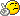# TGaxis problems

I am trying to use TGaxis to have on plot with seperate x and y axes, and a have a concern about the TGaxis constructor that takes a function name as input.

The code is found here:

http://root.cern.ch/root/html/TGaxis.html

```TF1 *f1=new TF1("f1","-x",-10,10); TGaxis *A1 = new TGaxis(0,2,10,2,"f1",510,"-"); A1->SetTitle("axis with decreasing values"); A1->Draw();```

It seems that what this should do is draw a new axis at y = 2 going from x = 0 -> 10 measured on the old axis and populate it with numbers that are the negative of the original axis.

Since the original axis goes from 0 -> 10, I would expect the new axis to go from 0 -> -10, but instead it draws an axis labeled 10 -> -10. It seems like it uses the domain of the TF1 rather than the values of the original axis to draw the new axis.

Maybe this solves other use cases, but I would think it would be much more natural for the new axis values to be calculated as a function of the old axis values. Is there a way to do this?

If You want to have two independent axes on the pad, You would need to come up with the transformation on the old axis values to the desired values on the other one…So it is actualy more natural/easier the way You are refering as the less natural one. Your case is easily solved by constructing the function with bounds 0,10…

Yes, what you describe seems to be the right way…but its not what ROOT is doing as far as I can tell.

If my original axis has 1, 2, 3, … and I want my new axis to have values -1, -2, -3 then my function should be: f(x) = -x

Similarly, if I wanted 1, 1/2, 1/3 then my function would be f(x) = 1/x.

But this is not the behavior ROOT provides.

``````TH2D *th = new TH2D("a","a",51, -0.25, 0.15, 51, 0.0, 0.11)
th -> Draw()
TF1 *f1 = new TF1("f1","1/(TMath::Sqrt(x))",1.0/TMath::Sqrt(0.03),1.0/TMath::Sqrt(0.11))
TGaxis *A1 = new TGaxis(-0.2, 0.03, -0.2, 0.11, "f1", 510,"+L")
A1 -> Draw()``````

This produces a plot with two axes, but a simple check with a calculator shows that the axes don’t give the desired behavior. 1/sqrt(0.06) = 4.08 not ~4.4 as the plot suggests.

Suggestions welcome!